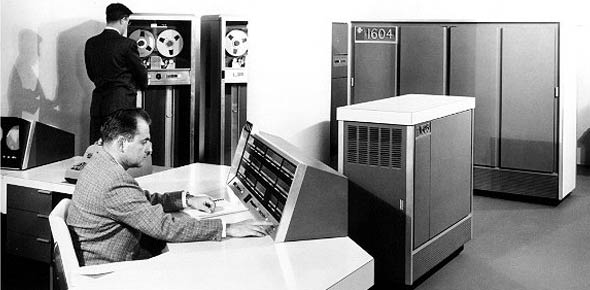# CDC 3C051 Pre-end Of Course (EOC) Preparation Exam

102 Questions | Attempts: 216
ShareSettingsPre-EOC test for CDC 3C051

• 1.
What is the first job the basic output system (BIOS) does at start-up?
• A.

Run memory byte count

• B.

Test for parity errors

• C.

Load instructions to random access memory (RAM)

• D.

Run the power-on self-test (POST)

• 2.
Which original component was very susceptible to damage from electrostatic discharge?
• A.

• B.

Basic input output system (BIOS)

• C.

Complementary metal-oxide semiconductor (CMOS)

• D.

Electrically erasable programmable read-only memory (EEPROM)

• 3.
Actively sampling the status of an external device by a client program is known as
• A.

Polling

• B.

Signaling

• C.

Processing

• D.

• 4.
Actively sampling the status of an external device by a client program is known as
• A.

Polling

• B.

Signaling

• C.

Processing

• D.

• 5.
What is defined as an asynchronous signal from a computer hardware device indicating th need for attention from a central processing unit (CPU) or a synchronous event in a software application program indicating a need for a change in execution?
• A.

Cache

• B.

Buffer

• C.

Interface

• D.

Interrupt

• 6.
What interrupt request (IRQ) line number is normally used for the floppy controller?
• A.

1

• B.

6

• C.

7

• D.

13

• E.

14

• 7.
The first 640 KB of memory is what division of memory?
• A.

Upper

• B.

Conventional

• C.

High

• D.

Extended

• 8.
What is defined as an asynchronous signal from a computer hardware device indicating th need for attention from a central processing unit (CPU) or a synchronous event in a software application program indicating a need for a change in execution?
• A.

Cache

• B.

Buffer

• C.

Interface

• D.

Interrupt

• 9.
The high memory area is how much of the extended memory area?
• A.

The first 64 KB

• B.

The first 256 KB

• C.

The first 1 MB

• D.

The first 2 MB

• 10.
What interrupt request (IRQ) line number is normally used for the floppy controller?
• A.

1

• B.

6

• C.

7

• D.

13

• E.

14

• 11.
Which type of RAM holds data without needing to be refreshed as long as power is supplied to them?
• A.

Dynamic random access memory (DRAM)

• B.

Synchronous dynamic random access memory (SDRAM)

• C.

Double data rate synchronous dynamic random access memory (DDR SDRAM)

• D.

Static random access memory (SRAM)

• 12.
The first 640 KB of memory is what division of memory?
• A.

Upper

• B.

Conventional

• C.

High

• D.

Extended

• 13.
Operating system (OS) nstructions and OS programs are processed into which memory space?
• A.

Conventional

• B.

Upper

• C.

High

• D.

Extended

• 14.
The high memory area is how much of the extended memory area?
• A.

The first 64 KB

• B.

The first 256 KB

• C.

The first 1 MB

• D.

The first 2 MB

• 15.
A Class I Electrostatic Discharge (ESD) item is senstive to what level of voltage?
• A.

Less than 1000 volts

• B.

Between 1,000 but less than 3,999 volts

• C.

More than 15,000 volts

• D.

Between 4,000 but less than 15,000 volts

• 16.
Which type of RAM holds data without needing to be refreshed as long as power is supplied to them?
• A.

Dynamic random access memory (DRAM)

• B.

Synchronous dynamic random access memory (SDRAM)

• C.

Double data rate synchronous dynamic random access memory (DDR SDRAM)

• D.

Static random access memory (SRAM)

• 17.
A Class II Electrostatic Discharge (ESD) item is senstive to what level of voltage?
• A.

Less than 1000 volts

• B.

Between 1,000 but less than 3,999 volts

• C.

More than 15,000 volts

• D.

Between 4,000 but less than 15,000 volts

• 18.
Operating system (OS) nstructions and OS programs are processed into which memory space?
• A.

Conventional

• B.

Upper

• C.

High

• D.

Extended

• 19.
A Class III Electrostatic Discharge (ESD) item is senstive to what level of voltage?
• A.

Less than 1000 volts

• B.

Between 1,000 but less than 3,999 volts

• C.

More than 15,000 volts

• D.

Between 4,000 but less than 15,000 volts

• 20.
A Class I Electrostatic Discharge (ESD) item is senstive to what level of voltage?
• A.

Less than 1000 volts

• B.

Between 1,000 but less than 3,999 volts

• C.

More than 15,000 volts

• D.

Between 4,000 but less than 15,000 volts

• 21.
Static electricity remains intact until it is
• A.

Absored

• B.

Dissolved

• C.

Discharged

• D.

Neutralized

• 22.
A Class II Electrostatic Discharge (ESD) item is senstive to what level of voltage?
• A.

Less than 1000 volts

• B.

Between 1,000 but less than 3,999 volts

• C.

More than 15,000 volts

• D.

Between 4,000 but less than 15,000 volts

• 23.
In which troubleshooting step would you determine which conditions are present?
• A.

Define the problem

• B.

Isolate the problem

• C.

Resolve the problem

• D.

Confirm the resolution

• 24.
A Class III Electrostatic Discharge (ESD) item is senstive to what level of voltage?
• A.

Less than 1000 volts

• B.

Between 1,000 but less than 3,999 volts

• C.

More than 15,000 volts

• D.

Between 4,000 but less than 15,000 volts

• 25.
In which troubleshooting step would you confirm a dilemma exists?
• A.

Define the problem

• B.

Isolate the problem

• C.

Resolve the problem

• D.

Confirm the resolution

## Related TopicsBack to top
×

Wait!
Here's an interesting quiz for you.